## Dividend Portfolios by Ian Kaplan December 2021 </h2>

### Introduction

The classic conservative investment portfolio often consists of approximately 40 percent stocks (perhaps a mix of stock ETFs) and 60 percent bonds (perhaps a US Treasury bond ETF). This type of portfolio is discussed in the All Weather Portfolio notebook.

The portfolios investigated in the All Weather Portfolio notebook have an annual return, on average, of approximately nine percent. These returns are highly volatile from year to year.

#### Interest Rate Risk

In an era of low interest rates (December 2021), with a possible interest rate increases in the future, an investment portfolio that consists mostly of bonds has interest rate risk.

When interest rates rise, the value of current bond holdings decrease as the bonds are discounted for the higher prevailing interest rate. The return paid by ETF bond funds at the time this was written were around 3 percent. This level of return does not compensate for the interest rate risk carried by a significant position in bonds.

Considering the interest rate risk of a 40 percent stock/60 percent bond portfolio, such portfolio is not, in fact, a conservative investment.

#### Market Risk

With the 2008 financial crisis and the COVID-19 pandemic, governments in the United States, Europe and Japan have embarked on fiscal policies that are referred to as "quantitative easing". This has resulted in interest rates that have been near zero. This has made many bond investments unattractive, causing huge investment flows into stock market.

The result is a stock market with high valuations and less room for growth, especially if interest rates rise.

The P/E ratio is a classic measure of any security's value, indicating how many years of profits (at the current rate) it takes to recoup an investment in the stock. The current S&P500 10-year P/E Ratio is 37.5. This is 89% above the modern-era market average of 19.6, putting the current P/E 2.3 standard deviations above the modern-era average. This suggests that the market is Strongly Overvalued.

https://www.currentmarketvaluation.com/models/price-earnings.php

#### Dividends

An alternative to the classic 40/60 stock/bond portfolio is a portfolio of dividend assets. A diversified portfolio that returns approximately 7% from dividends should have lower volatility, compared to a 40/60 stock/bond portfolio. The focus of a dividend portfolio is not capital appreciate of the assets (as would be the case with a stock portfolio), but dividend yield.

A dividend portfolio does have stock market risk, since all of the assets are either stocks, ETFs are closed-end funds. A market crash or downturn will have an effect on the face vaue of the portfolio. The face value of the portfolio is only an issue if the assets are sold. If the dividend portfolio continues to provide an attractive yield, it would be held in the long term. The face value of the portfolio could be expected to recover over time from a downturn.

### Actively Managed Funds

Actively managed stock funds have been widely criticized for their high fees and returns that often lag low cost index funds or ETFs.

A number of the assets in the portfolio explored by this notebook are actively managed. These actively managed funds yield more consistent and often higher dividend returns than a passive collections of dividend stocks in a low fee ETF.

### Dividend Yielding ETFs, Funds and Stocks

The table below summarizes the dividend yielding assets explored in this notebook. These assets were found from a number of sources, including an Australian friend who invests in the Australian stock market.

Yields are approximate since they change as the market price and the dividend amounts issued.

ASX is the Australian stock market. The dividends from the Australian stocks in the table below are "fully franked" which means that the taxes are paid by the issuer, which avoids tax withholding.

CEF: Closed End Fund

Almost half of the assets in the table below are involved with natural resources (e.g., iron, steel, oil, pipelines, etc...):

• FMG
• BHP
• RIO
• CEQP
• WMB
• XOM
Name market symbol Type Exchange Gross Yield Expense ratio Net yield Inception date
Fortescue Metals Group Ltd FMG Stock ASX 20% - - -
BHP Group Ltd BHP Stock NYSE 12% - - -
Rio Tinto Ltd RIO Stock NYSE 11% - - -
Crestwood Equity Partners LP CEQP Stock NYSE 9.61% - - -
Ares Capital Corporation ARCC Stock NASDAQ 8% - - -
Eaton Vance Limited Duration Income Fund EVV CEF NYSE 9.11% 1.91 7.2 May 30, 2003
PIMCO Corporate & Income Opportunity Fund PTY CEF NYSE 8% 1.09% 7% 12/27/2002
Nationwide Risk-Managed Income ETF NUSI ETF NYSE 7.59% 0.68% 6.91% 12/27/2002
Suncorp Group Ltd SUN Stock ASX 6.89 - - -
Westpac Banking Corporation WBK Stock NYSE 6.28 - - -
The Williams Companies, Inc. WMB Stock NYSE 6% - - -
Exxon Mobil Corporation XOM Stock NYSE 5.72 - - -
W. P. Carey Inc. WPC Stock NYSE 5.34 - - -
Blackrock Core Bond Trust BHK CEF NYSE 5.54 0.92 4.62 Nov 30, 2001

#### Notes

Australian stock quotes: https://www2.asx.com.au/markets/

Australian stock market quotes can also be obtained from Yahoo finance by appending the .AX suffix to the stock symbol.

Australian stocks can be purchased via the foreign stock "desk" at US brokerages.

#### NUSI ETF

From etf.com:

NUSI is actively managed portfolio of stocks included in the Nasdaq-100 Index and an options collar. Per index rules, the fund only invests in the top 100 largest by market cap, nonfinancial stocks listed on NASDAQ. A collar strategy involves selling or writing call options and buying put options, thus generating income to hedge some downside risk. The strategy seeks to generate high current income on a monthly basis from any dividends received from the underlying stock and the option premiums retained.

The NUSI hedging strategy seems to be successful. The market beta of the NUSI ETF is 0.44.

### Obtaining Dividend Data

Dividend data can be obtained via the yfinance tool. See https://pypi.org/project/yfinance/

In :
import yfinance as yf
import pandas as pd
from tabulate import tabulate
import matplotlib.pyplot as plt
import tempfile
from pathlib import Path
from typing import List
from datetime import datetime
from forex_python.converter import CurrencyRates

def get_dividend_data(symbol: str, file_name:str) -> pd.Series:
temp_root: str = tempfile.gettempdir() + '/'
file_path: str = temp_root + file_name
temp_file_path = Path(file_path)
file_size = 0
if temp_file_path.exists():
file_size = temp_file_path.stat().st_size

if file_size > 0:
dividend_data = pd.Series(dividend_data[dividend_data.columns])
else:
yfData = yf.Ticker(symbol)
dividend_data: pd.Series = pd.Series(yfData.dividends)
dividend_data.to_csv(file_path)
return dividend_data

def get_market_data(file_name: str,
data_col: str,
symbols: str,
data_source: str,
start_date: datetime,
end_date: datetime) -> pd.Series:
"""
file_name: the file name in the temp directory that will be used to store the data
data_col: the type of data - 'Adj Close', 'Close', 'High', 'Low', 'Open', Volume'
symbols: a list of symbols to fetch data for
data_source: yahoo, etc...
start_date: the start date for the time series
end_date: the end data for the time series
Returns: a Pandas DataFrame containing the data.

If a file of market data does not already exist in the temporary directory, fetch it from the
data_source.
"""
temp_root: str = tempfile.gettempdir() + '/'
file_path: str = temp_root + file_name
temp_file_path = Path(file_path)
file_size = 0
if temp_file_path.exists():
file_size = temp_file_path.stat().st_size

if file_size > 0:
close_data: pd.Series = close_data_df[close_data_df.columns]
else:
panel_data: pd.DataFrame = data.DataReader(symbols, data_source, start_date, end_date)
close_data: pd.Series = pd.Series(panel_data[data_col])
close_data.to_csv(file_path)
return close_data


### Historical dividend payments

In :
def get_dividend(symbol: str) -> pd.Series:
dividend_file = f'{symbol}_dividends'
dividends = get_dividend_data(symbol, dividend_file)
if (len(dividends) > 0):
div_date_series = pd.to_datetime( dividends.index )
dividends.index = div_date_series
return dividends

def get_dividend_return(symbol: str, dividends: pd.Series) -> pd.Series:
'''
This function returns the dividend return for a time series of
dividends.

The dividend return is the dividend returned at time t, divided
by the market asset price a time t.
'''
if len(dividends) > 0:
div_date_series = pd.to_datetime(dividends.index)
end_date = div_date_series[len(div_date_series) - 1]
end_year = end_date.year
end_month = end_date.month
start_date = datetime(end_year - 10, end_month, 1)
start_date = max(start_date, div_date_series)
data_source = 'yahoo'
close_file = f'{symbol}_close'
close_values = get_market_data(file_name=close_file,
data_col='Close',
symbols=symbol,
data_source=data_source,
start_date=start_date,
end_date=end_date)
close_date_series = pd.to_datetime(close_values.index)
close_ix = close_date_series.isin(div_date_series)
dividend_close = close_values[close_ix]
dividend_ix = div_date_series.isin(close_date_series)
percent_series = pd.Series(percent)
else:
percent_series = pd.Series([])
return percent_series

def get_yearly_return(dividend_ret: pd.Series) -> pd.Series:
'''
Return the yearly return for a set of dividend returns. The yearly
return is the sum, over the year period, of the dividend returns.
'''
if len(dividend_ret) > 0:
yearly_div: List = []
div_dates: List = []
div_value = dividend_ret
div_year = dividend_ret.index
for i in range(1, dividend_ret.shape):
if dividend_ret.index[i].year == div_year.year:
div_value = div_value + dividend_ret[i]
div_year = dividend_ret.index[i]
else:
yearly_div.append(div_value)
div_dates.append(div_year)
div_value = dividend_ret[i]
div_year = dividend_ret.index[i]
yearly_div.append(div_value)
div_dates.append(div_year)
yearly_div_series = pd.Series(yearly_div)
yearly_div_series.index = div_dates
else:
yearly_div_series = pd.Series([])
return yearly_div_series

def get_asset_yearly_ret(symbol: str) -> pd.Series:
dividends = get_dividend(symbol)
div_return = get_dividend_return(symbol, dividends)
yearly_ret = get_yearly_return(div_return)
return yearly_ret

symbols = ['FMG.AX', 'BHP', 'RIO', 'CEQP', 'ARCC', 'EVV', 'PTY',
'NUSI', 'SUN.AX', 'WBK', 'WMB', 'XOM', 'WPC', 'BHK']

expense = {'FMG.AX': 0, 'BHP': 0, 'RIO': 0, 'CEQP': 0, 'ARCC': 0,
'EVV': 0.0191, 'PTY': 0.0109, 'NUSI': 0.0068, 'SUN.AX': 0,
'WBK': 0, 'WMB': 0, 'XOM': 0, 'WPC': 0, 'BHK': 0.0092}

c = CurrencyRates()
aux_to_dollar = c.get_rate('AUD', 'USD')

exchange_adj = {'FMG.AX': aux_to_dollar, 'BHP': 1, 'RIO': 1, 'CEQP': 1, 'ARCC': 1,
'EVV': 1, 'PTY': 1, 'NUSI': 1, 'SUN.AX': aux_to_dollar,
'WBK': 1, 'WMB': 1, 'XOM': 1, 'WPC': 1, 'BHK': 1}

dividend_dict: dict = dict()
for sym in symbols:
yearly_ret = get_asset_yearly_ret(sym)
dividend_dict[sym] = yearly_ret

for sym in symbols:

def plot_bar(subplot, symbol, data, width) -> None:
title = f'{symbol} Yearly Dividend Yield'
subplot.set_ylabel('Percent')
subplot.set_title(title)
subplot.bar(data.index, data.values, width=width, color='blue')


### Yearly Dividend Return Plots

The plots below show the yearly dividend returns for each of the assets. If the asset has over ten years of history, only the last ten years are shown.

Return is calculated by adding up the dividend return percentages for the year. For example, if the asset has a price of 100 and a dividend of 5 in June and a price of 100 and a dividend of 6 in December, the yearly return will be 11 percent.

If the asset is an ETF or fund, the expense ratio is subtracted from the yearly return.

In :
trading_days = 253
i = 0
while i < num_assets - (num_assets % 2):
fig = plt.figure(figsize=(10, 6))
sym1 = keys[i]
sym2 = keys[i+1]
plt.show()
i = i + 2

if num_assets % 2 > 0:
i = num_assets - 1
fig = plt.figure(figsize=(5, 6))
sym = keys[i]
plt.show()

mean_return = dict()
mean_return[sym] = m

percent_sum = sum(list(mean_return.values()))
portfolio_percent = dict()
for sym in list(mean_return):
percent = round(mean_return[sym] / percent_sum, 4)
portfolio_percent[sym] = percent
portfolio_percent_df = pd.DataFrame( list(portfolio_percent.values()) )
portfolio_percent_df.index = list(portfolio_percent.keys())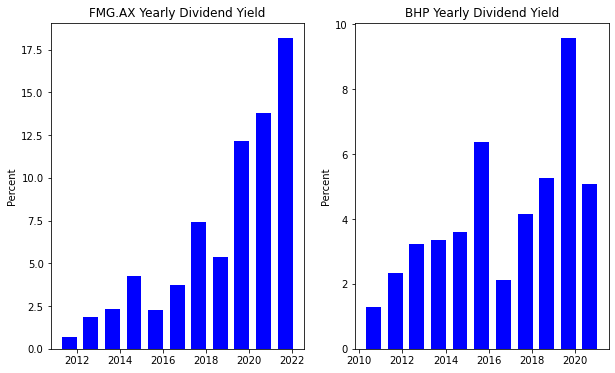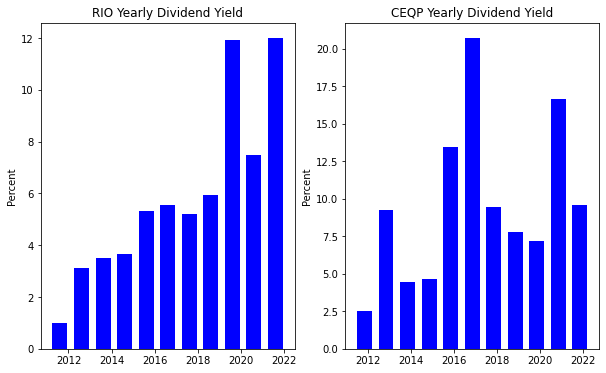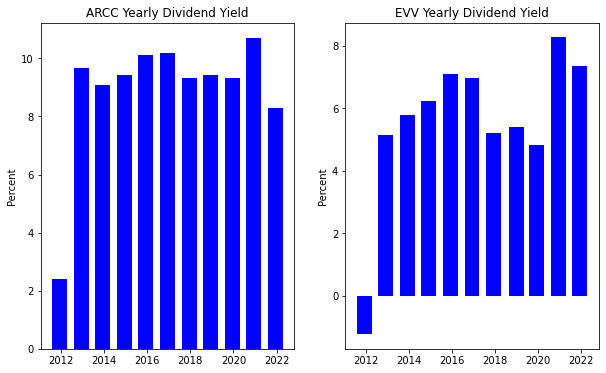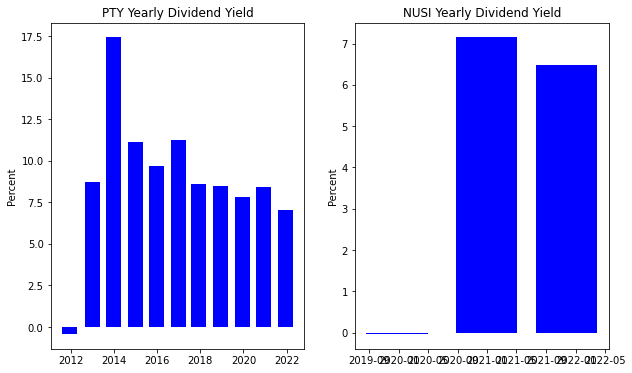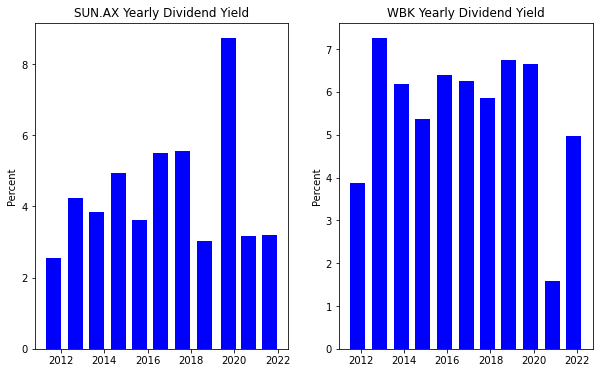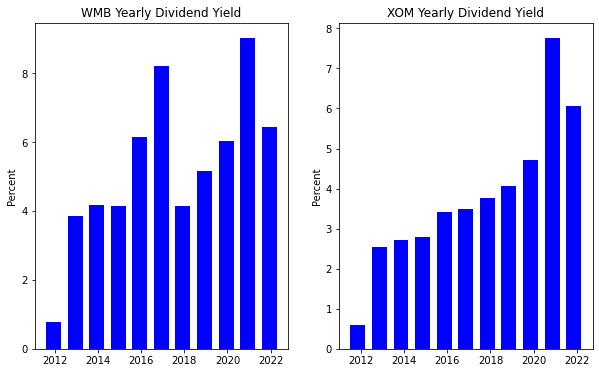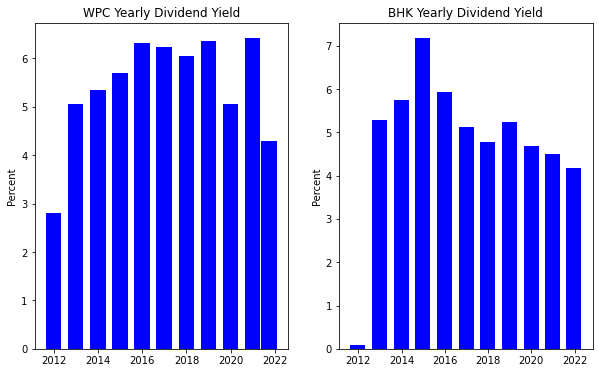### Portfolio Statistics

The percentage of the portfolio allocated for each asset is shown in the table below.

The following steps are used to calculate the asset percentages:

1. Calculate the mean yearly dividend return for each asset

The mean return is used instead of the median return because it will provide some bias for an asset that has large returns (for example, FMG).

2. Sum the mean returns
3. The portfolio percentage for each asset is the mean return of the asset divided by the sum of the mean returns.

This allocates a higher percentage of the portfolio to assets with high mean returns.

In :
print("Portfolio Percentage")
print(tabulate(portfolio_percent_df * 100, headers=['Symbol', 'Portfolio Percent'], tablefmt='fancy_grid'))

capital = 100000

capital_df = pd.DataFrame([capital])
print(tabulate(capital_df, headers=['', 'Capital (USD) invested in portfolio'], tablefmt='fancy_grid'))

allocation_df = portfolio_percent_df * capital

print("Fetching current stock prices...")
print()

prices_low = prices['Low']
prices_high = prices['High']
prices_mid = round((prices_low + prices_high) / 2, 2)

for sym in prices_mid.columns:

Portfolio Percentage
╒══════════╤═════════════════════╕
│ Symbol   │   Portfolio Percent │
╞══════════╪═════════════════════╡
│ FMG.AX   │                7.84 │
├──────────┼─────────────────────┤
│ BHP      │                5.05 │
├──────────┼─────────────────────┤
│ RIO      │                7.05 │
├──────────┼─────────────────────┤
│ CEQP     │               11.52 │
├──────────┼─────────────────────┤
│ ARCC     │               10.67 │
├──────────┼─────────────────────┤
│ EVV      │                6.66 │
├──────────┼─────────────────────┤
│ PTY      │               10.7  │
├──────────┼─────────────────────┤
│ NUSI     │                5.44 │
├──────────┼─────────────────────┤
│ SUN.AX   │                5.27 │
├──────────┼─────────────────────┤
│ WBK      │                6.67 │
├──────────┼─────────────────────┤
│ WMB      │                6.33 │
├──────────┼─────────────────────┤
│ XOM      │                4.57 │
├──────────┼─────────────────────┤
│ WPC      │                6.49 │
├──────────┼─────────────────────┤
│ BHK      │                5.75 │
╘══════════╧═════════════════════╛
╒════╤═══════════════════════════════════════╕
│    │   Capital (USD) invested in portfolio │
╞════╪═══════════════════════════════════════╡
│  0 │                                100000 │
╘════╧═══════════════════════════════════════╛
╒══════════╤═════════════════════╕
│ Symbol   │   Dollar Allocation │
╞══════════╪═════════════════════╡
│ FMG.AX   │                7840 │
├──────────┼─────────────────────┤
│ BHP      │                5050 │
├──────────┼─────────────────────┤
│ RIO      │                7050 │
├──────────┼─────────────────────┤
│ CEQP     │               11520 │
├──────────┼─────────────────────┤
│ ARCC     │               10670 │
├──────────┼─────────────────────┤
│ EVV      │                6660 │
├──────────┼─────────────────────┤
│ PTY      │               10700 │
├──────────┼─────────────────────┤
│ NUSI     │                5440 │
├──────────┼─────────────────────┤
│ SUN.AX   │                5270 │
├──────────┼─────────────────────┤
│ WBK      │                6670 │
├──────────┼─────────────────────┤
│ WMB      │                6330 │
├──────────┼─────────────────────┤
│ XOM      │                4570 │
├──────────┼─────────────────────┤
│ WPC      │                6490 │
├──────────┼─────────────────────┤
│ BHK      │                5750 │
╘══════════╧═════════════════════╛
Fetching current stock prices...
[*********************100%***********************]  14 of 14 completed



### Current Stock Prices

The stock prices returned by finance.yahoo.com for FMG.AX and SUN.AX are in Australian dollars. This stock price has been adjusted for the current US dollar to Australian dollar exchange rate.

In :
print(f"Share prices as of {prices_mid_adj.index}")

allocation_df_t = allocation_df.transpose()
shares_df = pd.DataFrame()
for sym in allocation_df_t.columns:

print(tabulate(shares_df.transpose(), headers=['Symbol', 'Number of Shares'], tablefmt='fancy_grid'))

invested_total = 0
for sym in shares_df.columns:
invested_total = invested_total + asset_val

invested_total_df = pd.DataFrame(invested_total)

portfolio_return_df = pd.DataFrame()
portfolio_percent_df_t = portfolio_percent_df.transpose()
for sym in portfolio_percent_df.index:

total_return = round(portfolio_return_df.values.sum(), 4) * 100
total_return_df = pd.DataFrame([total_return])

Share prices as of 2021-12-17 00:00:00
╒══════════╤═══════════════╕
│ Symbol   │   Share Price │
╞══════════╪═══════════════╡
│ ARCC     │       19.64   │
├──────────┼───────────────┤
│ BHK      │       16.44   │
├──────────┼───────────────┤
│ BHP      │       58.63   │
├──────────┼───────────────┤
│ CEQP     │       24.77   │
├──────────┼───────────────┤
│ EVV      │       12.9    │
├──────────┼───────────────┤
│ FMG.AX   │       13.5974 │
├──────────┼───────────────┤
│ NUSI     │       27.72   │
├──────────┼───────────────┤
│ PTY      │       16.42   │
├──────────┼───────────────┤
│ RIO      │       65.47   │
├──────────┼───────────────┤
│ SUN.AX   │        7.7587 │
├──────────┼───────────────┤
│ WBK      │       14.94   │
├──────────┼───────────────┤
│ WMB      │       25.73   │
├──────────┼───────────────┤
│ WPC      │       80.45   │
├──────────┼───────────────┤
│ XOM      │       60.48   │
╘══════════╧═══════════════╛
╒══════════╤════════════════════╕
│ Symbol   │   Number of Shares │
╞══════════╪════════════════════╡
│ FMG.AX   │                576 │
├──────────┼────────────────────┤
│ BHP      │                 86 │
├──────────┼────────────────────┤
│ RIO      │                107 │
├──────────┼────────────────────┤
│ CEQP     │                465 │
├──────────┼────────────────────┤
│ ARCC     │                543 │
├──────────┼────────────────────┤
│ EVV      │                516 │
├──────────┼────────────────────┤
│ PTY      │                651 │
├──────────┼────────────────────┤
│ NUSI     │                196 │
├──────────┼────────────────────┤
│ SUN.AX   │                679 │
├──────────┼────────────────────┤
│ WBK      │                446 │
├──────────┼────────────────────┤
│ WMB      │                246 │
├──────────┼────────────────────┤
│ XOM      │                 75 │
├──────────┼────────────────────┤
│ WPC      │                 80 │
├──────────┼────────────────────┤
│ BHK      │                349 │
╘══════════╧════════════════════╛
╒═════════════════════╤══════════════════╕
│                     │   Total Invested │
╞═════════════════════╪══════════════════╡
│ 2021-12-17 00:00:00 │          99811.6 │
╘═════════════════════╧══════════════════╛


### Portfolio Dividend Return

The estimate for the portfolio dividend return is the sum of the mean return for each asset times the percentage of that asset in the portfolio.

The mean return for each asset has the fund cost subtracted for ETFs and CEFs.

In :
print(tabulate(total_return_df, headers=['', 'Estimated Dividend Return'], tablefmt='fancy_grid'))

╒════╤═════════════════════════════╕
│    │   Estimated Dividend Return │
╞════╪═════════════════════════════╡
│  0 │                        6.51 │
╘════╧═════════════════════════════╛


The stock market over the last two years (2020 and 2021) has grown, on average, about 20 percent. The "conservative" portfolio, of 40% stocks and 60% bonds, described in the All Weather Portfolio notebook returns, on average, 9 percent. The return of the dividend portfolio is disappointing by comparison.

Such a comparatively low return only becomes attractive only if you hold the view that stock market growth will be low or negative in the next few years (e.g., after 2021) and that interest rates will rise, resulting in losses in bond portfolios.

## Data Issues

The information in this notebook, as the disclaimer below states, is for informational purposes only.

At the dawn of the Internet age, there was a saying "information wants to be free". Market data, however, costs money and free data, like that from finance.yahoo.com, may have more errors than curated commercial data sources.

This can particularly true for foreign dividends data. For example, the dividend yield reported for Suncorp Group (SUN.AX) by the Australian exchange is 6.86 percent. A complete list of dividends can be found on the Suncorp website.

As the table below shows, the dividends reported by finance.yahoo.com do not align with those reported on Suncorp's web site. The result is an under-estimation of Suncorp dividend returns.

Assuming that the return of Suncorp is, in fact, 6.86 percent it's percentage in the portfolio should be about the same as Westpack Banking Corp (WBK) with a dividend return of 6.28 percent. Because the dividend return of SUN.AX is under-estimated the percentage allocated in the portfolio is 1.4 percent less than WBK.

#### Dividends Reported on the Suncorp website and finance.yahoo.com

Sun Corp Date Amount finance.yahoo.com Date Amount
22 September 2021 0.40
22 September 2021 0.08 Aug 13, 2021 0.08
1 April 2021 0.26 Feb 15, 2021 0.26
21 October 2020 0.10 Aug 26, 2020 0.1
31 March 2020 0.26 Feb 19, 2020 0.26
25 September 2019 0.44 Sep 30, 2019 0.401648

Year Return
2019 8.73
2020 3.177
2021 3.186

#### Disclaimer

This notebook is not financial advice, investing advice, or tax advice. The information in this notebook is for informational and recreational purposes only. Investment products discussed (ETFs, mutual funds, etc.) are for illustrative purposes only. This is not a recommendation to buy, sell, or otherwise transact in any of the products mentioned. Do your own due diligence. Past performance does not guarantee future returns.

In :